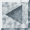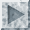The Primary Tasks of Data MiningThe two "high-level" primary goals of data mining, in practice, are prediction and description.

1. Prediction involves using some variables or fields in the database to predict unknown or future values of other variables of interest.
2. Description focuses on finding human-interpretable patterns describing the data.

The relative importance of prediction and description for particular data mining applications can vary considerably. However, in the context of KDD, description tends to be more important than prediction. This is in contrast to pattern recognition and machine learning applications (such as speech recognition) where prediction is often the primary goal of the KDD process.

The goals of prediction and description are achieved by using the following primary data mining tasks:

1. Classification is learning a function that maps (classifies) a data item into one of several predefined classes.
2. Regression is learning a function which maps a data item to a real-valued prediction variable.
3. Clustering is a common descriptive task where one seeks to identify a finite set of categories or clusters to describe the data.
• Closely related to clustering is the task of probability density estimation which consists of techniques for estimating, from data, the joint multi-variate probability density function of all of the variables/fields in the database.
4. Summarization involves methods for finding a compact description for a subset of data.
5. Dependency Modeling consists of finding a model which describes significant dependencies between variables.
Dependency models exist at two levels:
1. The structural level of the model specifies (often graphically) which variables are locally dependent on each other, and
2. The quantitative level of the model specifies the strengths of the dependencies using some numerical scale.
6. Change and Deviation Detection focuses on discovering the most significant changes in the data from previously measured or normative values.